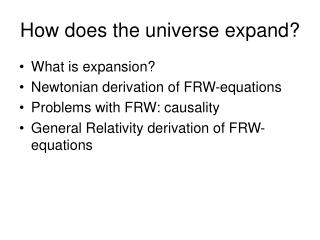DownloadDownload PresentationHow does the universe expand?

# How does the universe expand?

Download Presentation## How does the universe expand?

- - - - - - - - - - - - - - - - - - - - - - - - - - - E N D - - - - - - - - - - - - - - - - - - - - - - - - - - -
##### Presentation Transcript

1. How does the universe expand? • What is expansion? • Newtonian derivation of FRW-equations • Problems with FRW: causality • General Relativity derivation of FRW-equations

2. What is expansion?

3. Newtonian Derivation

4. FRW-equations

5. Special Relativity: 2 postulates: • The laws of physics are the same for all observers • The speed of light is equal to the same constant for all observers

6. Causality Space-time according to O2: The guy who is running from O1 to the TV Space-time according to O1: The guy who’s watching TV

7. The OPERA-experiment

8. GR-derivation of FRW-equations • Events given by 4-vectors • A tensor, like , is a generalised matrix • Metric tensor defines distances • Indices are raised/lowered by and it’s matrix inverse: • Energy momentum tensor is • Einstein’s equation (16 equations!) is:

9. Homogeneity & Isotropy demand: Flat space, k = 0 and sum = 180° Spherical space, k = +1, sum > 180° Hyperbolical space, k = -1, sum < 180°

10. The calculation • Put this into Einstein’s equation (16!): • Mathematicagives: only 2 independent equations: k = 0 Flat space k = +1 Spherical space k = -1 Hyperbolical space

11. Conclusion: How Does the universe expand? Homogeneity & Isotropy: Gravity: Equation of state: Special relativity demands v < c if causality should hold Does not apply for distant galaxies, since communication is impossible